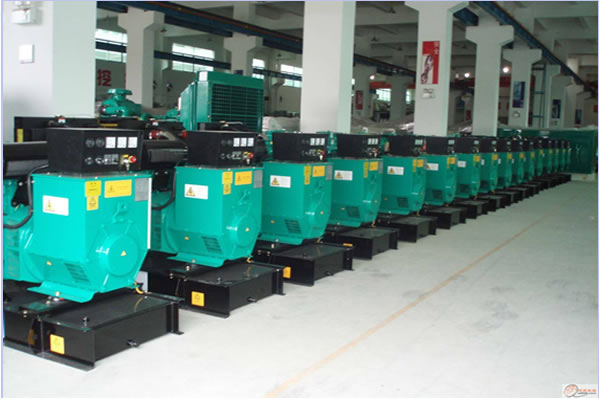186-8973-5688

# 玉柴发电机组如何计算耗油量

2019-05-2230kw玉柴柴油发电机组油耗量=6.3公斤(kg)=7.8升(L)
45kw玉柴柴油发电机组油耗量=9.45公斤(kg)=11.84升(L)
50kw玉柴柴油发电机组油耗量=10.5公斤(kg)=13.1升(L)
75kw玉柴柴油发电机组油耗量=15.7公斤(kg)=19.7升(L)
100kw柴油发电机组油耗量=21公斤(kg)=26.25升(L)
150kw柴油发电机组油耗量=31.5公斤(kg)=39.4升(L)
200kw柴油发电机组油耗量=40公斤(kg)=50升(L)
250kw柴油发电机组油耗量=52.5公斤(kg)=65.6升(L)
300kw柴油发电机组油耗量=63公斤(kg)=78.75升(L)
350kw柴油发电机组油耗量=73.5公斤(kg)=91.8升(L)
400kw柴油发电机组油耗量=84.00公斤(kg)=105.00升(L)
450kw柴油发电机组油耗量=94.50公斤(kg)=118.00升(L)
500kw柴油发电机组油耗量=105.00公斤(kg)=131.20升(L)

 阿里康明斯柴油发电机组厂家销售办事处: 拉萨康明斯柴油发电机组厂家销售办事处: 那曲康明斯柴油发电机组厂家销售办事处: 日喀则康明斯柴油发电机组厂家销售办事处: 西藏康明斯柴油发电机组厂家销售办事处: 昌都康明斯柴油发电机组厂家销售办事处: 山南康明斯柴油发电机组厂家销售办事处: 林芝康明斯柴油发电机组厂家销售办事处: